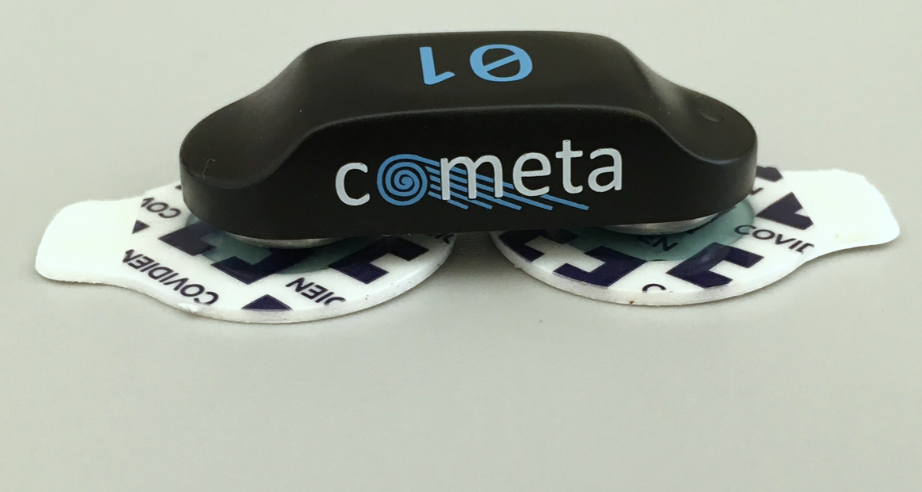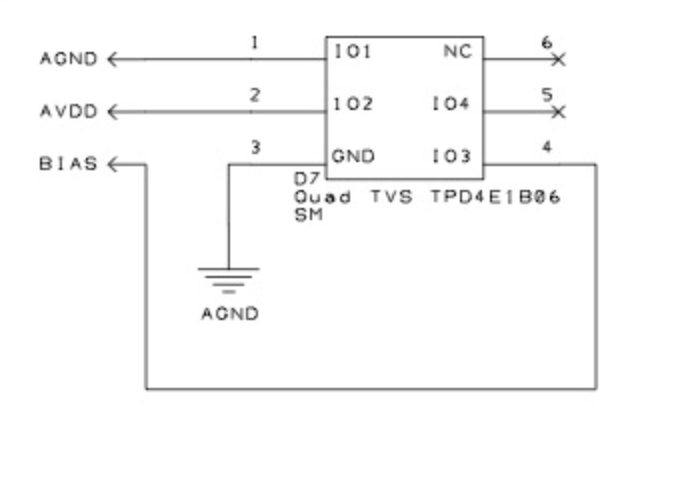# How are EMG channels calculated?

edited January 25

I see a lot of different electrode systems. OpenBCI uses electrodes to calculate each EMG channel and a common reference ground. Is the signal for each channel (abs(electrode1 - electrode2-ground))? I have also seen systems where there are two electrodes for each channel but no common ground. In these systems is there a separate ground for each system ? How are the channel value calculated?
Devices like the myo armband use three electrodes for each channel, with a separate ground for each channel, is the signal for each channel (abs(electrode1 - electrode2-ground))?

Which system is the best?

• BCI, hi.

EMG electrodes are almost always placed in differential pairs.

This differs from EEG, which uses a single common reference electrode. Both EEG and EMG also use a single ground electrode, which can be placed anywhere on the body.

William

• Note a few of the diagrams at the link above, confuse the labeling of the ground electrode, and label it as 'reference'. This is just confusing and they actually mean the single ground electrode. There is no common reference in EMG.

• How is the signal for the channel calculated relative to the differential electrodes and ground?

• EEG and EMG are both differential. They subtract the plus from the minus electrodes.

• Ground is not involved at all in the channel value calculation. Ground is only used as an input to the high gain differential amplifier to keep it centered and reduce noise.

• edited January 25

Thanks! So in EEG when there is just one electrode from each channel, it's just the measure of that electrode? What is the ground used for in EEG ?

• EEG is differential, just like EMG. In EEG, one single electrode (the 'reference' or SRB2 for Cyton), acts as the other polarity for subtracting from the channel electrode.

Just as in EMG, for EEG, the ground electrode is not involved in the subtraction. It's just used for reducing noise.

• edited January 25

What about in EMG systems that only have two electrodes with no ground or common reference? Or is one of the electrodes the ground and the other the reference like in EEG system? Like https://www.cometasystems.com/products/picoemg• In such devices, one of the electrodes also acts as ground. But the key is that EMG and EEG are always differential, subtracting voltages between two electrodes.

• Thank you! Just to clarify, while both EEG and EMG are differential systems, in EMG it is differential between a pair of electrodes, typically a common ground. In the example of Cometa above, the two electrodes form a differential pair, but there is no common ground, so one of the electrodes is both part of the differential pair and also the ground?
Whereas in EEG there is both a common ground and a common reference, and the differential is taken across the electrode and common reference. The ground in both EEG and EMG is not involved directly in the channel calculation, but serves as the input to the high gain differential amplifier to keep it centered and reduce noise.

Did I get this right or am I misunderstanding something?

• One way to think of this is as a difference of two differences:
Active electrode voltage = active wire voltage - ground wire voltage (removes some noise and DC offset)
Reference electrode voltage = reference wire voltage - ground wire voltage
Active voltage graphed on screen numbers = active electrode - reference electrode voltages

• Bill, thanks.

... in EMG it is differential between a pair of electrodes, typically a common ground. In the example of Cometa above, the two electrodes form a differential pair, but there is no common ground, so one of the electrodes is both part of the differential pair and also the ground?

The ground is just present for noise purposes. I would not use the term 'common ground'. The voltage difference is between the differential pair being measured. In EEG and EMG really only one ground connection is needed. With two terminal devices like the Cometa, one electrode serves dual purpose as ground. But I would not call it 'common ground'.

• edited January 25

Thank you!
Are there any studies comparing these different systems? Which system -- a single common ground several differential pairs, a separate ground for each differential pair, and no specific electrode just for ground -- is best?

• Only one ground connection is really needed. Multiple ground connections I don't believe would serve much purpose. The Cometa has a ground connection.

• Hi! Thank you for explaining this, I have one more question.
As you said there is no "reference" in EMG, it is actually ground. In the ADS1299 what is the ground input?

• As you said there is no "reference" in EMG, it is actually ground. In the ADS1299 what is the ground input?

Hi. No, I did not say "no reference in EMG". Each channel has its own plus and minus connections, two wires per channel. So you can think of one wire as the reference and the other is being measured. Biosignal amps subtract these two plus and minus signals to get the differential result.

ADS1299 uses the 'Bias' pin, which is treated the same as Ground on other amps. Only one ground connection is needed for the entire body. As mentioned previously, "The ground in both EEG and EMG is not involved directly in the channel calculation, but serves as the input to the high gain differential amplifier to keep it centered and reduce noise."

Regards, William

• Thank you! Is this Bias In or Bias Ref that acts as the ground?

• Both pins labeled Bias (top and bottom) are the same 'Bias' connection to the ADS1299.

• edited March 19What are the inputs labeled 4,5,6 referring to? Is the bias in the image the same as the Bias pin in the cyton?
The ADS1299 does not have a single Bias pin, it has BIASIN, BIAS REF, BIAS OUT, BIAS INV which one is the Ground?
Thank you!

• edited March 19

This section of the Cyton schematic, contains multiple TVS (Transient Voltage Suppressor) chips. A TVS is like a "lightning arrestor" for electronics. Any high voltage static discharges, for example from a subject in wintertime picking up static from a wool rug, are shorted to ground and discharged safely. Rather than frying the electronics chip input pins.

• I see, that makes sense
Which pin on the on the ADS1299 is Ground? BIASIN, BIAS REF, BIAS OUT or BIAS INV?

• Or are none of those pins Ground individually, and is the Cyton Bias pin the Ground but the ADS1299 itself does not have a Ground equivalent?

• edited March 19

You should consult the ADS1299 data sheet from TI. For this chip, 'Bias' can be considered both an input and an output, connected to the subject. On the Cyton schematic, I believe 'BIAS_DRV' signal is a direct connection to the Bias header pin.

Do you understand that in most bio amplifers, especially EEG, the Ground (Bias), is considered a 'driven ground' and injects a small 180 degree out of phase mains frequency? This is to slightly counter the huge mains voltage induced in EEG electrode cables. Thus Bias needs to both sense this common mode voltage, AND inject against it.

ADS1299 DOES have the AGND, which is analog ground. But this is not connected to subject.

William